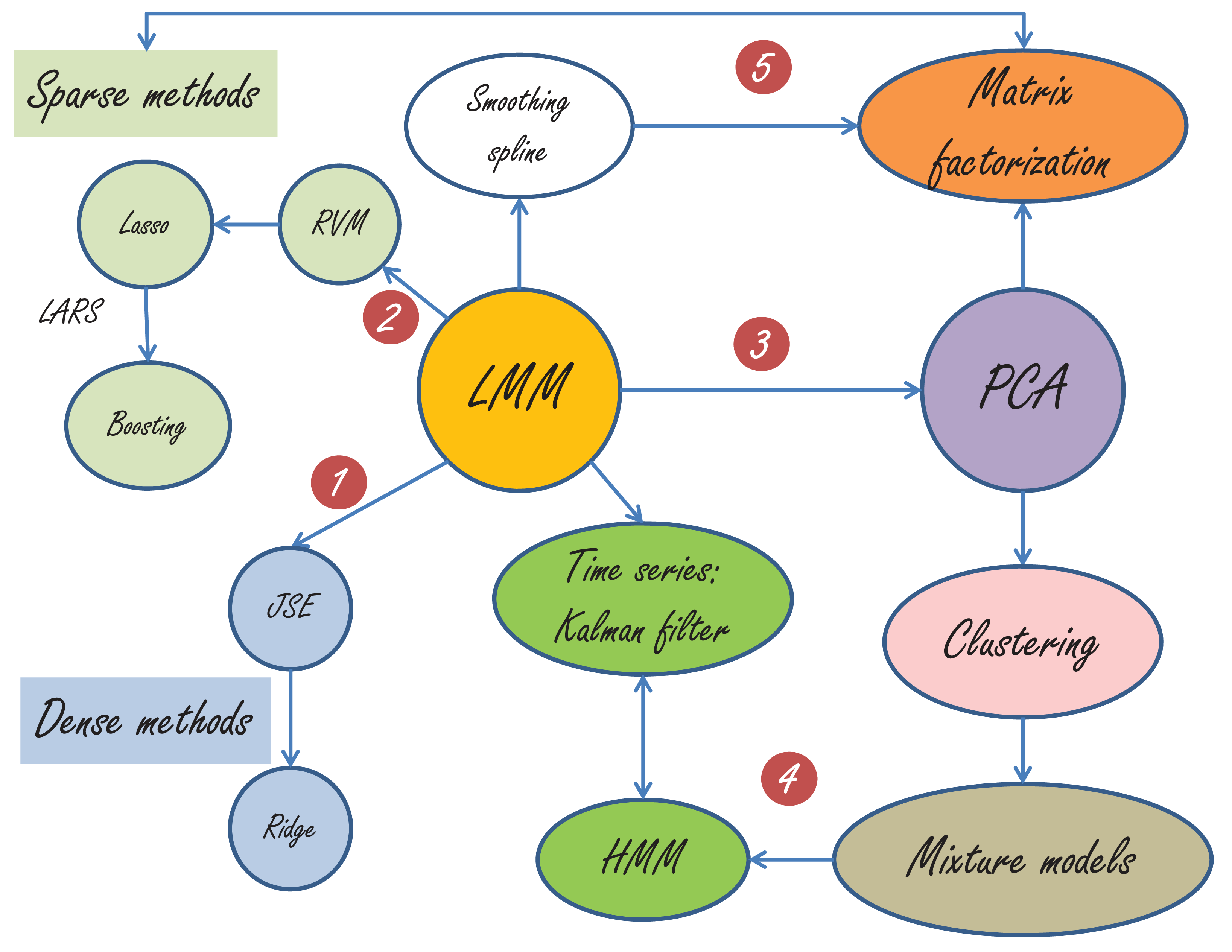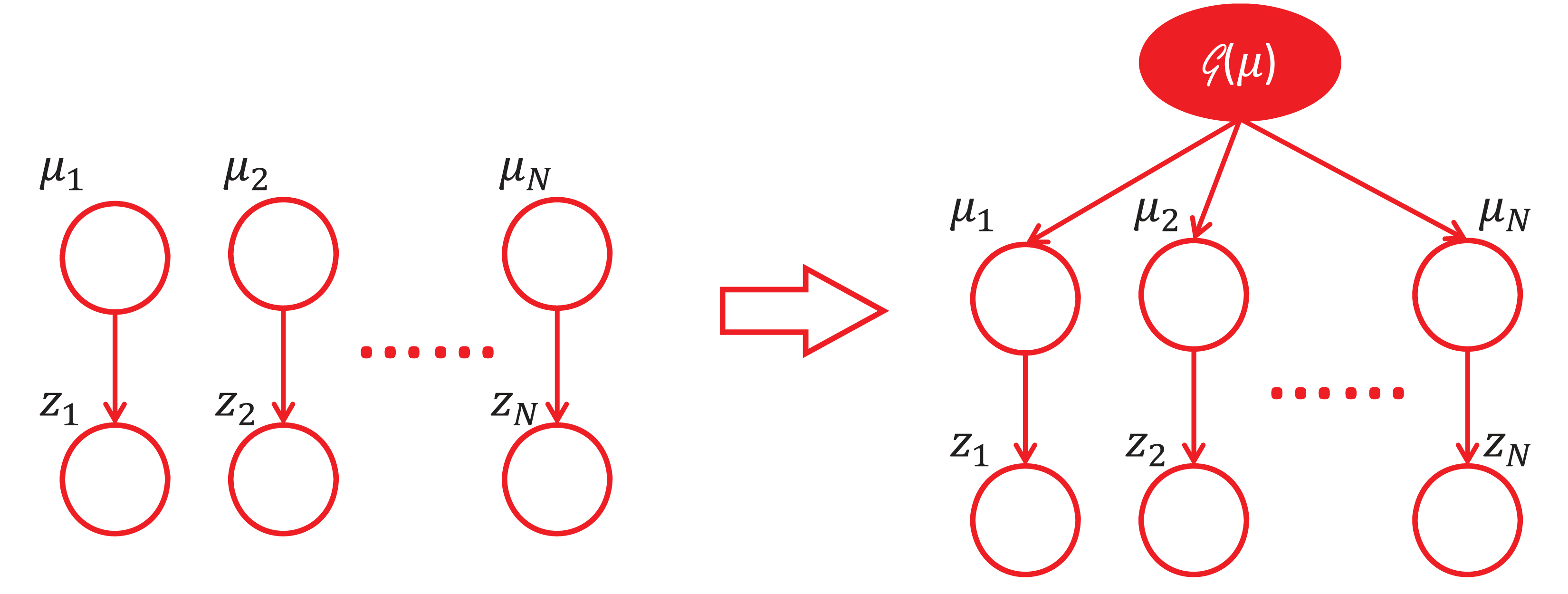2010年，夏。

# 2 从此醉

\begin{equation} \begin{aligned} \mathbf{y}&=\mathbf{X}\mathbf{\beta} + \mathbf{Zu}+\mathbf{e},\\ \mathbf{u}&\sim \mathcal{N}(\mathbf{0},\sigma^2_u\mathbf{I}),\\ \mathbf{e}&\sim\mathcal{N}(\mathbf{0},\sigma^2_e\mathbf{I})\\ \end{aligned} \end{equation}

$$\mathbf{y}=\mathbf{X}\mathbf{\beta} + \mathbf{e}, \quad \mathbf{e} \sim \mathcal{N}(\mathbf{0},\sigma^2_e\mathbf{I}),$$

\begin{aligned} \mathbf{y}&= \mathbf{Zu}+\mathbf{e},\\ \mathbf{u}&\sim \mathcal{N}(\mathbf{0},\sigma^2_u\mathbf{I}),\\ \mathbf{e}&\sim\mathcal{N}(\mathbf{0},\sigma^2_e\mathbf{I}), \end{aligned}

$$\min_{\mathbf{u}}\|\mathbf{y}-\mathbf{Z}\mathbf{u}\|^2+\lambda\|\mathbf{u}\|^2,$$

$$\mathbf{y}\sim\mathcal{N}(\mathbf{X}\mathbf{\beta},\mathbf{ZZ}^T \sigma^2_{u} + \sigma^2_{e} \mathbf{I}).$$

$$h^2=\frac{p{\sigma}^2_{u}}{p{\sigma}^2_{u}+{\sigma}^2_{e}},$$

# 3 水榭听香，指点群豪戏

“脚步不能达到的地方，眼光可以到达。”抬望眼，满城尽是LMM，如下图所示。由于篇幅所限，我只能简单地介绍一部分。LMM与很多经典方法的关系图

\begin{aligned} \mathbf{y}&= \sum_j \mathbf{Z}_j u_j+\mathbf{e},\\ u_j&\sim \mathcal{N}(0,\sigma^2_{u_j}),\\ \mathbf{e}&\sim\mathcal{N}(\mathbf{0},\sigma^2_e\mathbf{I}), \end{aligned}

RVM与LMM的差别是：RVM允许每个随机效应$u_j$有自己的方差$\sigma^2_{u_j}$，而LMM中所有的随机效应(random effects)具有同样的$\sigma^2_{u}$。 从RVM 到Lasso(Least Absolute Shrinkage and Selection Operator)，只需要假设$\{\sigma^2_{u_j}\}$来自指数分布，详情参见Bayesian Lasso。Lasso，LARS和Boosting已经成为统计学与计算科学史上的一段佳话，最好的文献当然是Efron教授的Least Angle Regression，喜欢看故事的童鞋可以看我的《统计学习那些事》。

In closing these few remarks, I cannot resist paraphrasing I.J. Good’s memorable aphorism: ‘To a Bayesian, all things are Bayesian.’ How does ‘To a non-Bayesian, all things are BLUPs’ sound as a summary of this fine paper?

# 4 杏子林中，商略平生義

“眼光不能到达的地方，精神可以飞到。”

“随机还是非随机？”是一个问题，甚至是一个哲学问题。或许，我们参一生也参不透这道难题。爱因斯坦说:“上帝不玩骰子。”然而，麦克斯韦却说：“这个世界真正的逻辑就是概率的计算。” 电影《美丽心灵》的纳什也在追问“到底什么才是真正的逻辑”。最后他在获得诺奖时说：“ 我一直以来都坚信数字，不管是方程还是逻辑都引导我们去思考。但是在如此追求了一生后，我问自己：‘逻辑到底是什么？谁决定原由？’我的探索让我从形而下到形而上，最后到了妄想症，就这样来回走了一趟。在事业上我有了重大突破，在生命中我也找到了最重要的人：只有在这种神秘的爱情方程中，才能找到逻辑或原由来。”这是我听到的最美的答案。

$$\hat{\mathbf{\mu}}_{ML} = \mathbf{z},\quad\hat{\mathbf{\mu}}_{JS} = \left( 1- \frac{N-2}{||\mathbf{z}||^2}\right)\mathbf{z}.$$# 5 须倾英雄泪

James Watson在他的《双螺旋》一书的序言中写道：“科学的发现很少会像门外汉所想像的那样，按照直截了当、合乎逻辑的方式进行。事实上，科学的进步（有时是倒退）往往是人为事件。在这些事件中，人性以及文化传统都起着巨大的作用。”

“庾信平生最萧瑟，暮年诗赋动江关。”这是张益唐教授为“孪生素数猜想”作出巨大贡献后接受采访时引用的诗句。听罢，令人感慨万千。

“胡汉恩仇”，须倾英雄泪。

1. BLUE 和 BLUP 分别表示 Best Linear Unbiased Estimate （最佳线性无偏估计）和 Best Linear Unbiased Prediction （最佳线性无偏预测） ↩︎